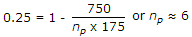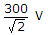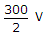# Electronics and Communication Engineering - Power Electronics

### Exercise :: Power Electronics - Section 2

16.

A single phase half wave controlled rectifier circuit has an R-L load. A freewheeling diode is also in the circuit. When freewheeling diode is conducting the SCR

 A. is forward biased B. is reverse biased C. may be forward biased or reverse biased D. forward biased initially but reverse biased afterwards

Explanation:

Free wheeling diode is forward biased only when SCR is reverse biased.

17.

An SBS can conduct is one direction only.

 A. True B. False

Explanation:

It is a bilateral switch.

18.

A 750 A circuit uses thyristor of current rating 175 A. If derating is 25%, the number of thyristors in parallel is

 A. 3 B. 4 C. 6 D. 10

Explanation:.

19.

Assertion (A): An inverter does not require forced commutation

Reason (R): A series inverter is a forced commutation inverter.

 A. Both A and R are correct and R is correct explanation of A B. Both A and R correct but R is not correct explanation of A C. A is correct but R is wrong D. A is wrong but R is correct

Explanation:

Some inverters require forced commutation.

20.

In a single phase full wave converter (M - 2 connection), the rms transformer voltage is 300 V across each half of secondary. The PIV for each thyristor is

 A. 300 V B.C. 6002 V D.